### Ce cours a été révisé!

Pour une expérience d'apprentissage plus agréable, nous vous recommandons d'étudier la version mobile relogée de ce cours.

Emmenez-moi au cours révisé.

- or -

Continue studying this course

# Inférence de données ; signification de la signification statistique ; interprétation des valeurs de p

• Notes d'étude
• Révisions du sujet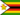Zivanai G. 0 0 Statistical significance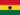Peter Awuni A. 0 0 What does the P stand for?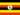Odongo M. 0 0 Psychology -> Inferring from data; meaning of statistical significance; interpretation of p values Inferring from data; meaning of statistical significance; interpretation of p values This is when statements or conclusions are drawn from the results obtained in an experiment. Inferences are made of the effect the IV has on the DV. Statistical significance This is a way of using statistical tests to determine the probability that an observed difference in the results is due to chance. If the difference is statistically significant, it is improbable that the difference was caused by chance; therefore, the hypothesis would be supported. The most commonly used significance levels are .05 and .01. If you decide before calculating your statistical tests that the .05 significance level is to be used, this means you will accept as a real difference only one that is so large that it could have occurred by chance only 5 times in 100 (p less than .05). If the .01 significance level is selected, then the difference can be expected to occur only 1 time in 100 by chance (p less than .01).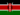Dominic M. 0 0 What margin can be said not to support the quess?Dominic M. 0 0 if the difference is significant and improbable that it was caused by chance,what then would support the hypothesis?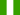Enemchukwu E. 0 0 This is a statement or conclusions are drawn from the results obtained in experiments.Linda T S. 0 0 The most commonly used significance levels are .05 and .01. If you decide before calculating your statistical tests that the .05 significance level is to be used, this means you will accept as a real difference only one that is so large that it could have occurred by chance only 5 times in 100 (p less than .05). If the .01 significance level is selected, then the difference can be expected to occur only 1 time in 100 by chance (p less than .01).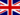Mike L. 0 0 Does a smaller p value indicate a more accurate outcome, how is p value decided initially ?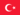Hassan O. 0 0 NiceEmmanuel O. 0 0 The IV and DV can it be separable when carrying out statistics?